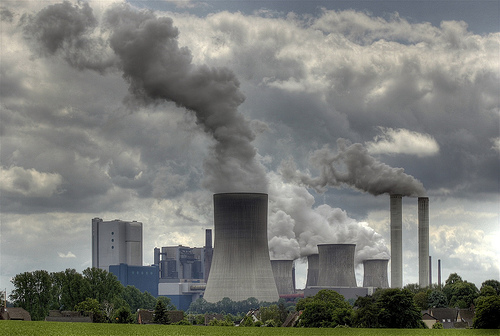# Environmental Chemistry

Chemistry Level 2The $\ce{pH}$ of a substance is the concentration $[\ce{H}^+]$, measured in moles of $\ce{H} ^+$ per liter of substance. It is given by the formula $\ce{pH} = \log_{10} \frac {1}{[\ce{H}^+]}$.

Find the concentration in moles per liter of hydrogen ion in a liter of acid rain that has a $\ce{pH}$ of 4.5.

×